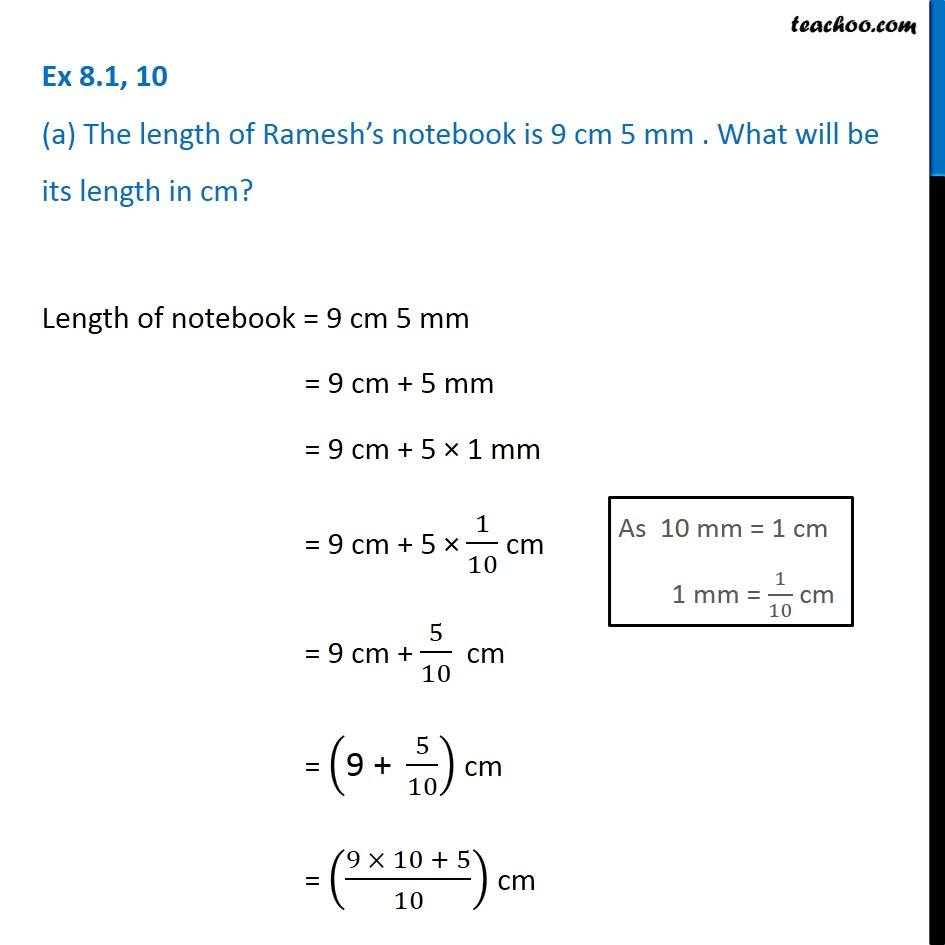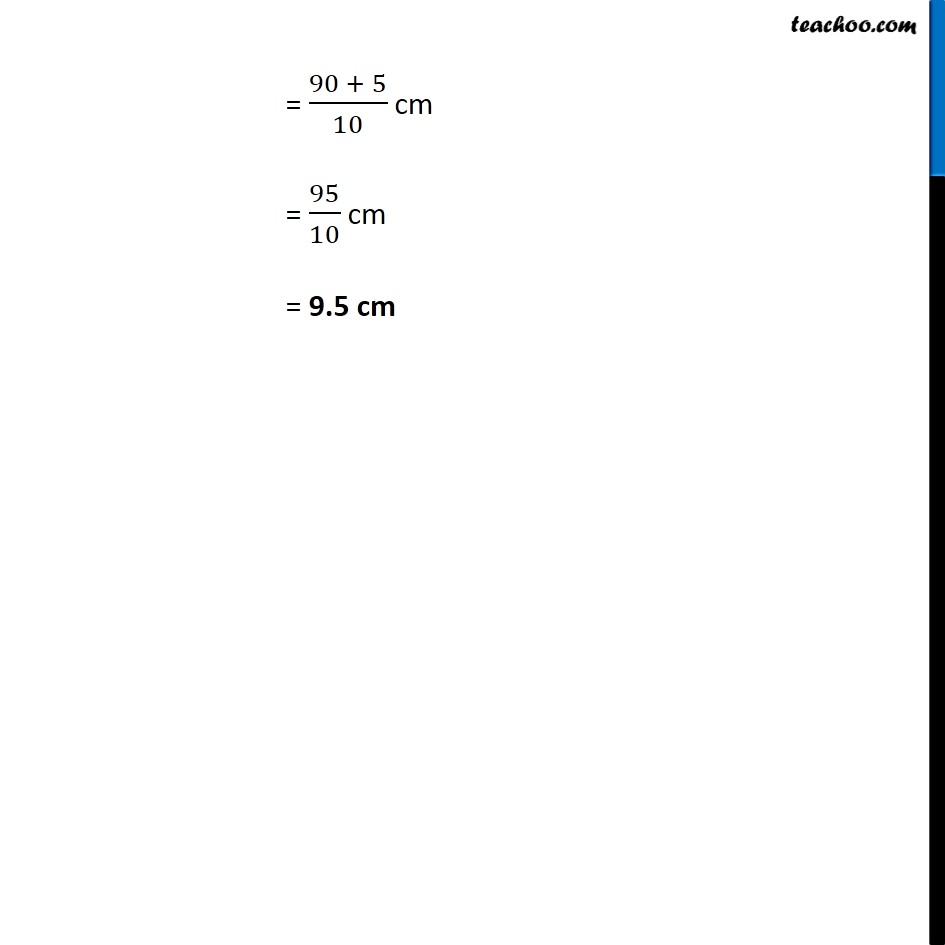Using Decimals - Converting to mm, cm, km, kg, rupees

Chapter 8 Class 6 Decimals
Concept wiseLearn in your speed, with individual attention - Teachoo Maths 1-on-1 Class

### Transcript

Question 10 (a) The length of Ramesh’s notebook is 9 cm 5 mm . What will be its length in cm? Length of notebook = 9 cm 5 mm = 9 cm + 5 mm = 9 cm + 5 × 1 mm = 9 cm + 5 × 1/10 cm = 9 cm + 5/10 cm = ("9 + " 5/10) cm = ((9 × 10 + 5)/10) cm = (90 + 5)/10 cm = 95/10 cm = 9.5 cm